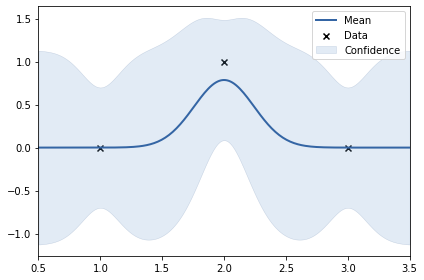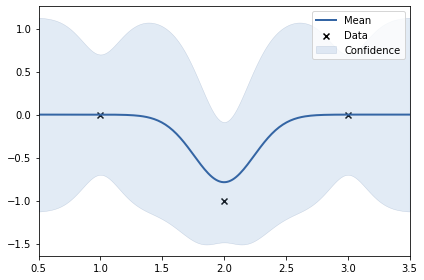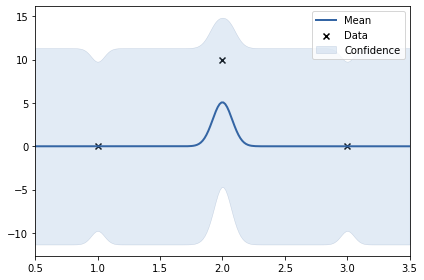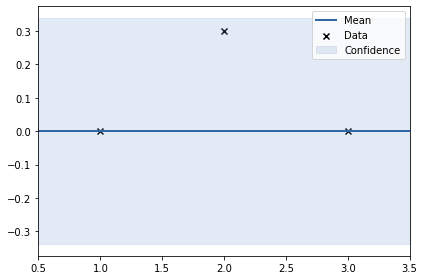# Understanding Kernels in Gaussian Processes Regression

Using GPy and some interactive visualisations for understanding GPR and applying on a real world data set
ML
Author

Nipun Batra

Published

June 26, 2020

### Disclaimer

This blog post is forked from GPSS 2019 Lab 1. This is produced only for educational purposes. All credit goes to the GPSS organisers.

# Support for maths
import numpy as np
# Plotting tools
from matplotlib import pyplot as plt
# we use the following for plotting figures in jupyter
%matplotlib inline

import warnings
warnings.filterwarnings('ignore')

# GPy: Gaussian processes library
import GPy
from IPython.display import display

### Covariance functions, aka kernels

We will define a covariance function, from hereon referred to as a kernel, using GPy. The most commonly used kernel in machine learning is the Gaussian-form radial basis function (RBF) kernel. It is also commonly referred to as the exponentiated quadratic or squared exponential kernel – all are equivalent.

The definition of the (1-dimensional) RBF kernel has a Gaussian-form, defined as:

$\kappa_\mathrm{rbf}(x,x') = \sigma^2\exp\left(-\frac{(x-x')^2}{2\mathscr{l}^2}\right)$

It has two parameters, described as the variance, $$\sigma^2$$ and the lengthscale $$\mathscr{l}$$.

In GPy, we define our kernels using the input dimension as the first argument, in the simplest case input_dim=1 for 1-dimensional regression. We can also explicitly define the parameters, but for now we will use the default values:

# Create a 1-D RBF kernel with default parameters
k = GPy.kern.RBF(lengthscale=0.5, input_dim=1, variance=4)
# Preview the kernel's parameters
k
rbf. valueconstraintspriors
variance 4.0 +ve
lengthscale 0.5 +ve
fig, ax = plt.subplots()
from matplotlib.animation import FuncAnimation
from matplotlib import rc
ls = [0.0005, 0.05, 0.25, 0.5, 1., 2., 4.]

X = np.linspace(0.,1.,500)# 500 points evenly spaced over [0,1]
X = X[:,None]
mu = np.zeros((500))

def update(iteration):
ax.cla()
k = GPy.kern.RBF(1)
k.lengthscale = ls[iteration]
# Calculate the new covariance function at k(x,0)
C = k.K(X,X)
Z = np.random.multivariate_normal(mu,C,40)
for i in range(40):
ax.plot(X[:],Z[i,:],color='k',alpha=0.2)
ax.set_title("$\kappa_{rbf}(x,x')$\nLength scale = %s" %k.lengthscale);
ax.set_ylim((-4, 4))

num_iterations = len(ls)
anim = FuncAnimation(fig, update, frames=np.arange(0, num_iterations-1, 1), interval=500)
plt.close()

rc('animation', html='jshtml')
anim

### In the animation above, as you increase the length scale, the learnt functions keep getting smoother.

fig, ax = plt.subplots()
from matplotlib.animation import FuncAnimation
from matplotlib import rc
var = [0.0005, 0.05, 0.25, 0.5, 1., 2., 4., 9.]

X = np.linspace(0.,1.,500)# 500 points evenly spaced over [0,1]
X = X[:,None]
mu = np.zeros((500))

def update(iteration):
ax.cla()
k = GPy.kern.RBF(1)
k.variance = var[iteration]
# Calculate the new covariance function at k(x,0)
C = k.K(X,X)
Z = np.random.multivariate_normal(mu,C,40)
for i in range(40):
ax.plot(X[:],Z[i,:],color='k',alpha=0.2)
ax.set_title("$\kappa_{rbf}(x,x')$\nVariance = %s" %k.variance);
ax.set_ylim((-4, 4))

num_iterations = len(ls)
anim = FuncAnimation(fig, update, frames=np.arange(0, num_iterations-1, 1), interval=500)
plt.close()

rc('animation', html='jshtml')
anim

### In the animation above, as you increase the variance, the scale of values increases.

X1 = np.array([1, 2, 3]).reshape(-1, 1)

y1 = np.array([0, 1, 0]).reshape(-1, 1)
y2 = np.array([0, -1, 0]).reshape(-1, 1)
y3 = np.array([0, 10, 0]).reshape(-1, 1)
y4 = np.array([0, 0.3, 0]).reshape(-1, 1)
k = GPy.kern.RBF(lengthscale=0.5, input_dim=1, variance=4)

m = GPy.models.GPRegression(X1, y1, k)
#m.Gaussian_noise = 0.0
m.optimize()
print(k)
m.plot();
  rbf.         |                value  |  constraints  |  priors
variance     |    0.262031485550043  |      +ve      |
lengthscale  |  0.24277532672486218  |      +ve      |k = GPy.kern.RBF(lengthscale=0.5, input_dim=1, variance=4)

m = GPy.models.GPRegression(X1, y2, k)
#m.Gaussian_noise = 0.0
m.optimize()
print(k)
m.plot();
  rbf.         |                value  |  constraints  |  priors
variance     |    0.262031485550043  |      +ve      |
lengthscale  |  0.24277532672486218  |      +ve      |### In the above two examples, the y values are: 0, 1, 0 and 0, -1, 0. This shows smoothness. Thus, length scale can be big (0.24)

k = GPy.kern.RBF(lengthscale=0.5, input_dim=1, variance=4)

m = GPy.models.GPRegression(X1, y3, k)
#m.Gaussian_noise = 0.0
m.optimize()
print(k)
m.plot();
  rbf.         |                value  |  constraints  |  priors
variance     |   16.918792970578004  |      +ve      |
lengthscale  |  0.07805339389352635  |      +ve      |### In the above example, the y values are: 0, 10, 0. The data set is not smooth. Thus, length scale learnt uis very small (0.24). Noise variance of RBF kernel also increased to accomodate the 10.

k = GPy.kern.RBF(lengthscale=0.5, input_dim=1, variance=4)

m = GPy.models.GPRegression(X1, y4, k)
#m.Gaussian_noise = 0.0
m.optimize()
print(k)
m.plot();
  rbf.         |                 value  |  constraints  |  priors
variance     |  5.90821963086592e-06  |      +ve      |
lengthscale  |     2.163452641925496  |      +ve      |